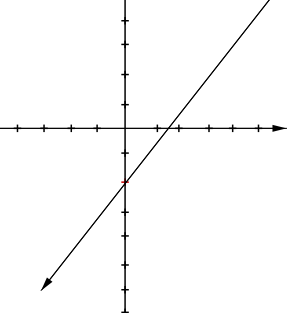### Home > GC > Chapter 1 > Lesson 1.2.3 > Problem1-76

1-76.

On graph paper, graph the line through the point $(0, −2)$ with slope $\frac { 4 } { 3 }$.1. Write the equation of the line.

$y=\frac{4}{3}x-2$

2. Translate the graph of the line up $4$ and to the right $3$ units. What is the result? Write the equation for the resulting line.

The resulting line coincides with the original line.

3. Now translate the original graph down $5$ units. What is the result? Write the equation for the resulting line.

The image is parallel.

$y=\frac { 4 } { 3 }x − 7$